Next: Wave Propagation Through Inhomogeneous Up: Waves in Cold Plasmas Previous: Parallel Wave Propagation

# Perpendicular Wave Propagation

Let us now consider wave propagation, at arbitrary frequencies, perpendicular to the equilibrium magnetic field. When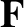, the eigenmode equation (490) simplifies to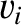(545)

One obvious way of solving this equation is to have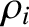, or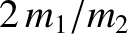(546)

with the eigenvector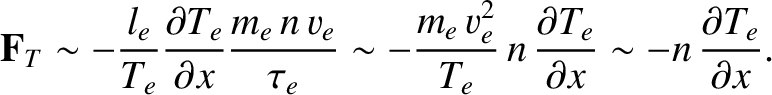. Since the wave-vector now points in the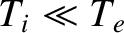-direction, this is clearly a transverse wave polarized with its electric field parallel to the equilibrium magnetic field. Particle motions are along the magnetic field, so the mode dynamics are completely unaffected by this field. Thus, the wave is identical to the electromagnetic plasma wave found previously in an unmagnetized plasma. This wave is known as the ordinary, or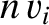-, mode.

The other solution to Eq. (545) is obtained by setting the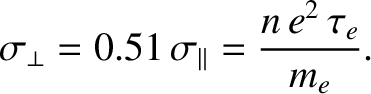determinant involving the- and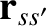- components of the electric field to zero. The dispersion relation reduces to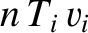(547)

with the associated eigenvector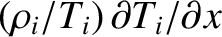.

Let us, first of all, search for the cutoff frequencies, at which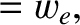goes to zero. According to Eq. (547), these frequencies are the roots ofand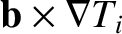. In fact, we have already solved these equations (recall that cutoff frequencies do not depend on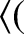). There are two cutoff frequencies,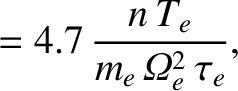and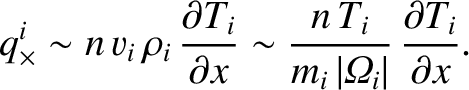, which are specified by Eqs. (541) and (544), respectively.

Let us, next, search for the resonant frequencies, at whichgoes to infinity. According to Eq. (547), the resonant frequencies are solutions of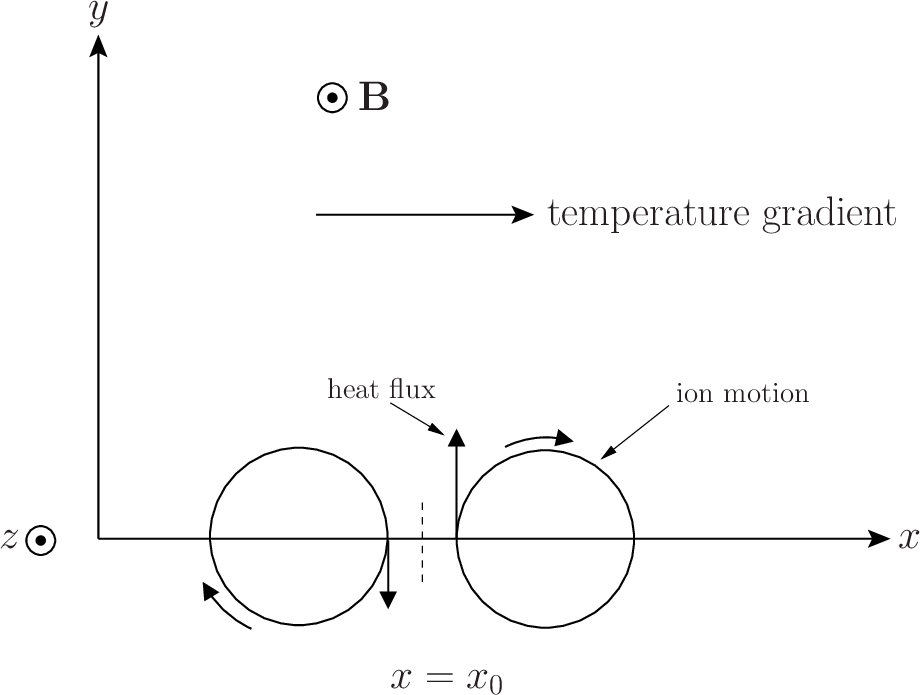(548)

The roots of this equations can be obtained as follows. First, we note that if the first two terms are equated to zero, we obtain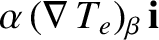, where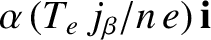(549)

If this frequency is substituted into the third term, the result is far less than unity. We conclude that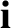is a good approximation to one of the roots of Eq. (548). To obtain the second root, we make use of the fact that the product of the square of the roots is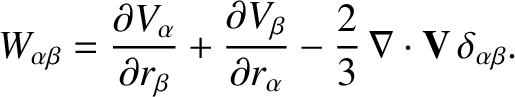(550)

We, thus, obtain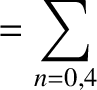, where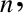(551)

The first resonant frequency,, is greater than the electron cyclotron or plasma frequencies, and is called the upper hybrid frequency. The second resonant frequency,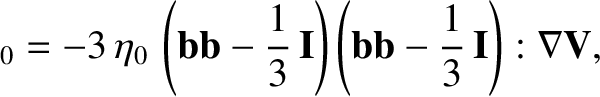, lies between the electron and ion cyclotron frequencies, and is called the lower hybrid frequency.

Unfortunately, there is no simple explanation of the origins of the two hybrid resonances in terms of the motions of individual particles.

At low frequencies, the mode in question reverts to the compressional-Alfvén wave discussed previously. Note that the shear-Alfvén wave does not propagate perpendicular to the magnetic field.

Using the above information, and the easily demonstrated fact that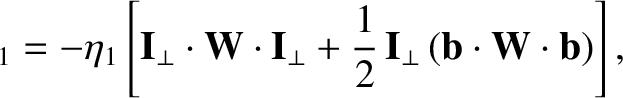(552)

we can deduce that the dispersion curve for the mode in question takes the form sketched in Fig. 14. The lowest frequency branch corresponds to the compressional-Alfvén wave. The other two branches constitute the extraordinary, or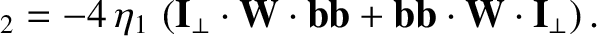-, wave. The upper branch is basically a linearly polarized (in the-direction) electromagnetic wave, somewhat modified by the presence of the plasma. This branch corresponds to a wave which propagates in the absence of an equilibrium magnetic field. The lowest branch corresponds to a wave which does not propagate in the absence of an equilibrium field. Finally, the middle branch corresponds to a wave which converts into an electrostatic plasma wave in the absence of an equilibrium magnetic field.Wave propagation at oblique angles is generally more complicated than propagation parallel or perpendicular to the equilibrium magnetic field, but does not involve any new physical effects.Next: Wave Propagation Through Inhomogeneous Up: Waves in Cold Plasmas Previous: Parallel Wave Propagation
Richard Fitzpatrick 2011-03-31